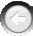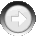﻿ CalcOrderArrayPath

# CalcOrderArrayPath

Navigation:  »No topics above this level«

# CalcOrderArrayPathCalcOrderArrayPath(NumOfPoints As Integer, OrderArray As Integer, Xarray As Single,Yarray As Single, Path As Single)   Purpose:  This function returns the path length of a closed tour going through a set of points, with each point being visited only once.  The function is used by the Traveling Salesman Problem example to speed up the calculation of the fitness function, which is much faster in a DLL than in Visual Basic.  This function may also be used in any application where fast calculation of a similar function is required.  Additional explanations may be found in Chapter 7, Programming Combinatorial Problems, the TSP Program section.   Arguments:   NumOfPoints is the number of points in two dimensional space which must be visited only once during a closed tour of the points.  This is an input parameter.  In the Traveling Salesman example, the NumOfPoints is equivalent to the number of cities visited by the salesman.   OrderArray is a one dimensional array of integers.  This is an input parameter, so it must be created and initialized in your application program.  The length of OrderArray must be equal to NumOfPoints.  The OrderArray must contain the city numbers in the order in which they are visited during the tour.  For example, if NumOfPoints = 5, then the OrderArray can look like {3,2,1,5,4}, or {2,5,1,3,4}, or {5,4,1,2,3}, and so on.   Xarray and Yarray are one dimensional arrays of X- and Y-coordinates, respectively, of all points.  These are input parameters, so these two single-precision arrays must be created and initialized in your application program.  The length of both arrays must be equal to NumOfPoints.   Path is the variable where the total distance length will be stored, so this is an output parameter.   Example: i = CalcOrderArrayPath(NumOfCities%, OrderArray(0), Xarray(0), Yarray(0), Path!)   This example calculates the length of the closed tour.  The number of cities is specified in NumOfCities. OrderArray contains the order of visitation, and city coordinates are in Xarray and Yarray.  The path length is returned in the Path variable.  Note that the first four parameters must be initialized by the user before calling this function.   Related Functions:  none.